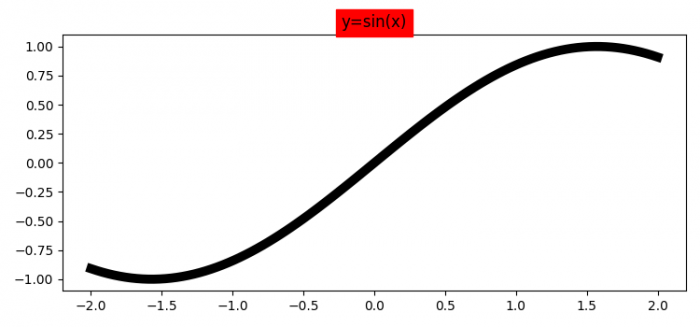# How do I make the width of the title box span the entire plot in Matplotlib?

To make width of title box span the entire plot in matplotlib, we can take the following steps

• Set the figure size and adjust the padding between and around the subplots.
• Create x and y data points using numpy.
• Plot x and y data points using plot() method, with color=black and linewidth=7.
• Get the current axes using gca() method.
• Set the title of of the plot.
• Return the bbox patch using get_bbox_patch() method
• To display the figure, use show() method.

## Example

import numpy as np
from matplotlib import pyplot as plt
plt.rcParams["figure.figsize"] = [7.50, 3.50]
plt.rcParams["figure.autolayout"] = True
x = np.linspace(-2, 2, 100)
y = np.sin(x)
plt.plot(x, y, c='black', lw=7)
ax = plt.gca()
title = ax.set_title("y=sin(x)", backgroundcolor='red')
bb = title.get_bbox_patch()
plt.show()

## Output